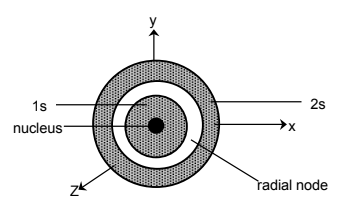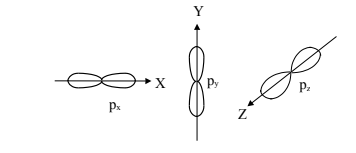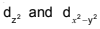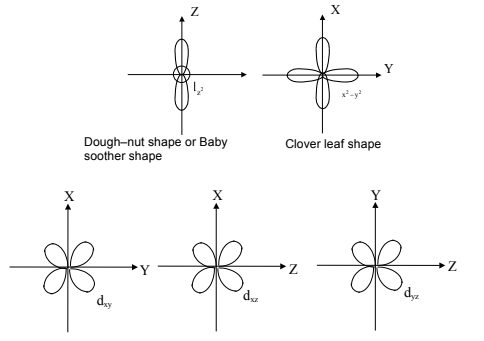# Shapes and size of orbitals

An orbital is the region of space around the nucleus within which the probability of finding an electron of given energy is maximum (90–95%). The shape of this region (electron cloud) gives the shape of the orbital. It is basically determined by the azimuthal quantum number l, while the orientation of orbital depends on the magnetic quantum number (m). Let us now see the shapes of orbitals in the various subshells.

s–orbitals: These orbitals are spherical and symmetrical about the nucleus. The probability of finding the electron is maximum near the nucleus and keep on decreasing as the distance from the nucleus increases. There is vacant space between two successive s–orbitals known as radial node. But there is no radial node for 1s orbital since it is starting from the nucleus.

The size of the orbital depends upon the value of principal quantum number(n). Greater the value of n, larger is the size of the orbital. Therefore, 2s–orbital is larger than 1s orbital but both of them are non-directional and spherically symmetrical in shape.p–orbitals (l =1): The probability of finding the p–electron is maximum in two lobes on the opposite sides of the nucleus. This gives rise to a dumb–bell shape for the p–orbital. For p–orbital l = 1. Hence, m = –1, 0, +1. Thus, p–orbital have three different orientations. These are designated as px,py & pz depending upon whether the density of electron is maximum along the x y and z axis respectively. As they are not spherically symmetrical, they have directional character. The two lobes of p–orbitals are separated by a nodal plane, where the probability of finding electron is zero.The three p-orbitals belonging to a particular energy shell have equal energies and are called degenerate orbitals.

d–orbitals (l =2): For d–orbitals, l =2. Hence m=–2,–1,0,+1,+2. Thus there are 5 d orbitals. They have relatively complex geometry. Out of the five orbitals, the three (dxy, dyz,dzx) project in between the axis and the other twolie along the axis.Post By : Mamta Singh 11 Jan, 2020 1699 views Chemistry